# Decompose flat footprints (structuring elements)#

Many footprints (structuring elements) can be decomposed into an equivalent series of smaller structuring elements. The term “flat” refers to footprints that only contain values of 0 or 1 (i.e., all methods in `skimage.morphology.footprints`). Binary dilation operations have an associative and distributive property that often allows decomposition into an equivalent series of smaller footprints. Most often this is done to provide a performance benefit.

As a concrete example, dilation with a square footprint of shape (15, 15) is equivalent to dilation with a rectangle of shape (15, 1) followed by another dilation with a rectangle of shape (1, 15). It is also equivalent to 7 consecutive dilations with a square footprint of shape (3, 3).

There are many possible decompositions and which one performs best may be architecture-dependent.

scikit-image currently provides two forms of automated decomposition. For the cases of `square`, `rectangle` and `cube` footprints, there is an option for a “separable” decomposition (size > 1 along only one axis at a time).

There is no separable decomposition into 1D operations for some other symmetric convex shapes, e.g., `diamond`, `octahedron` and `octagon`. However, it is possible to provide a “sequence” decomposition based on a series of small footprints of shape `(3,) * ndim`.

For simplicity of implementation, all decompositions shown below use only odd-sized footprints with their origin located at the center of the footprint.

•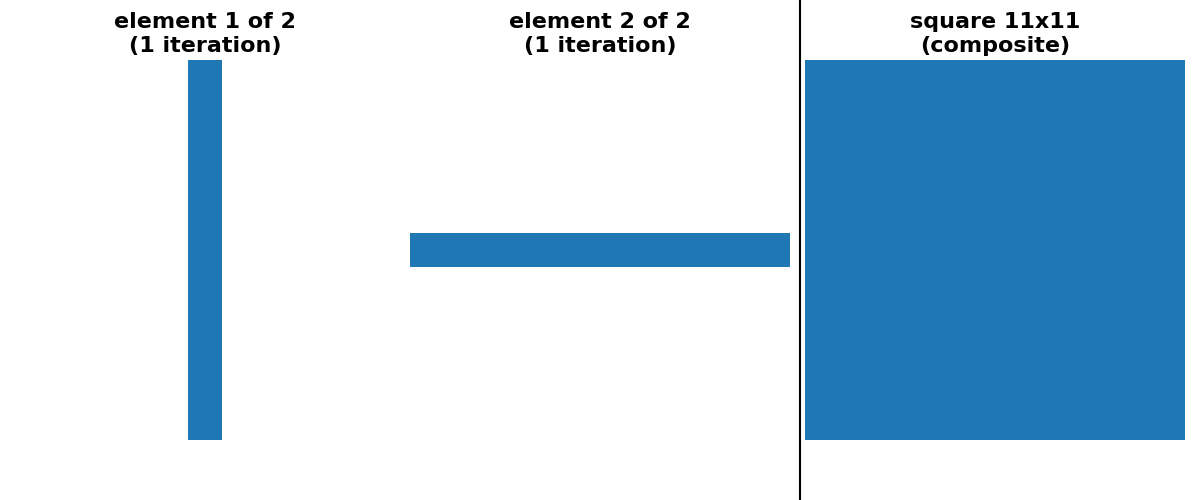•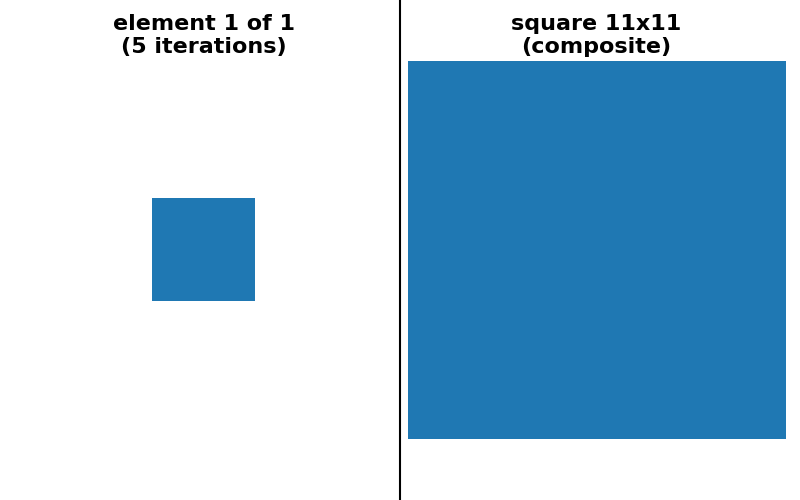•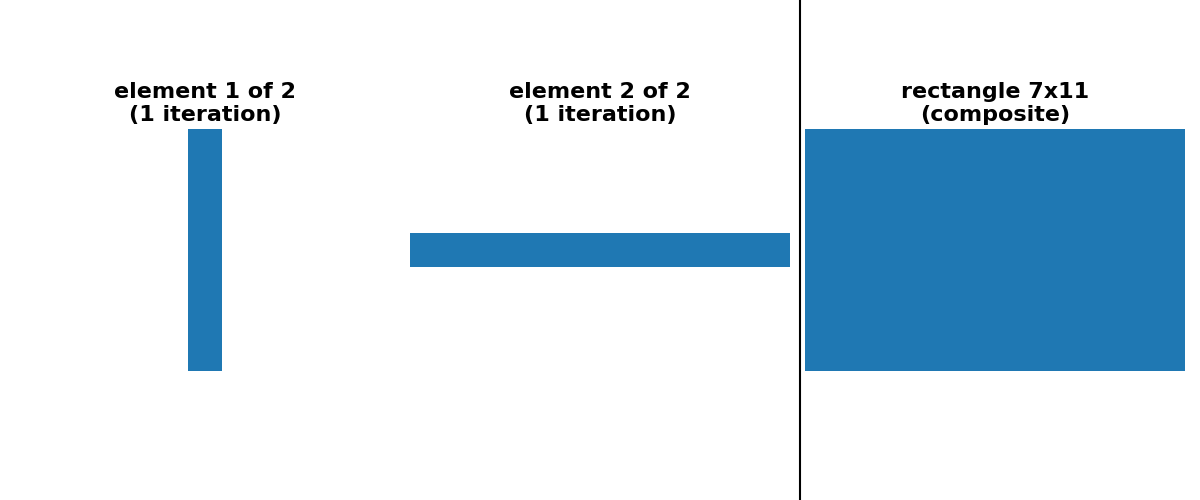•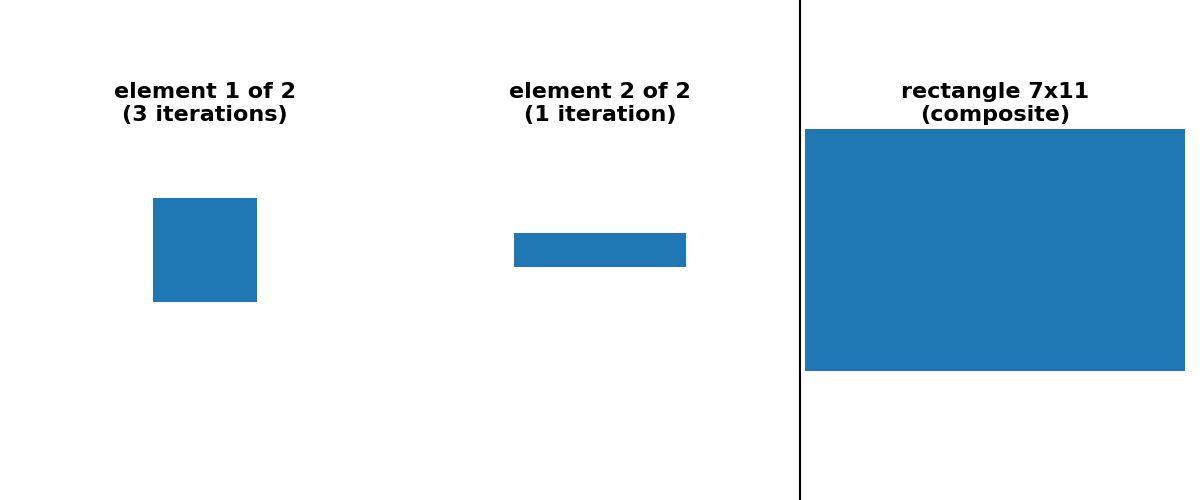•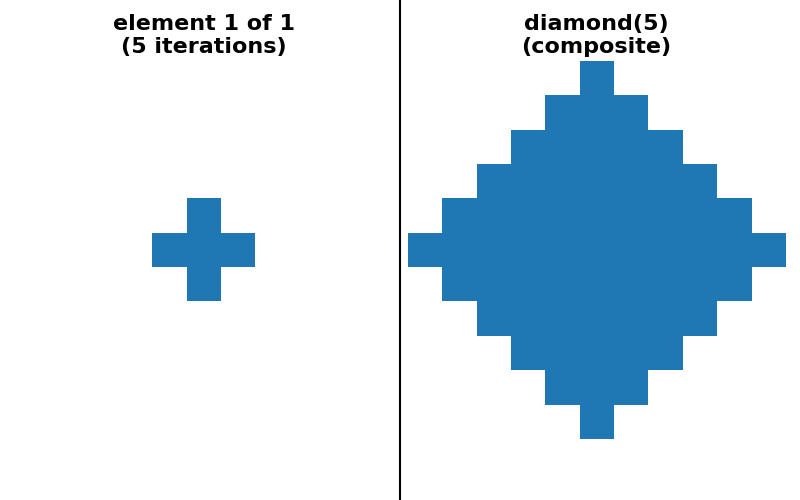•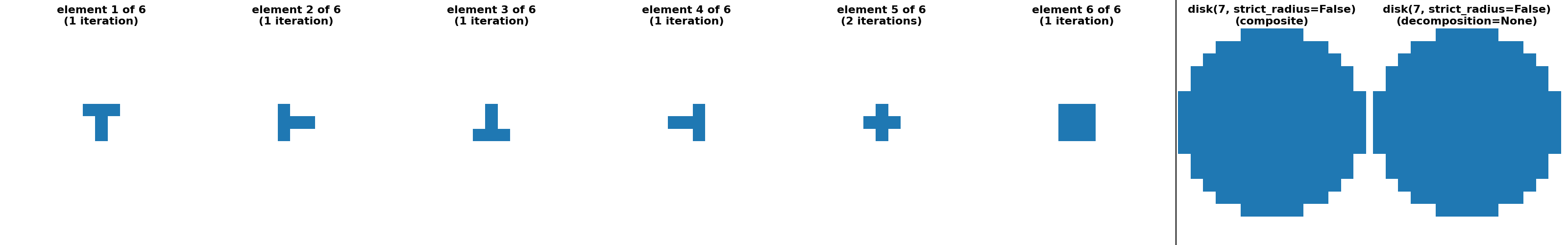•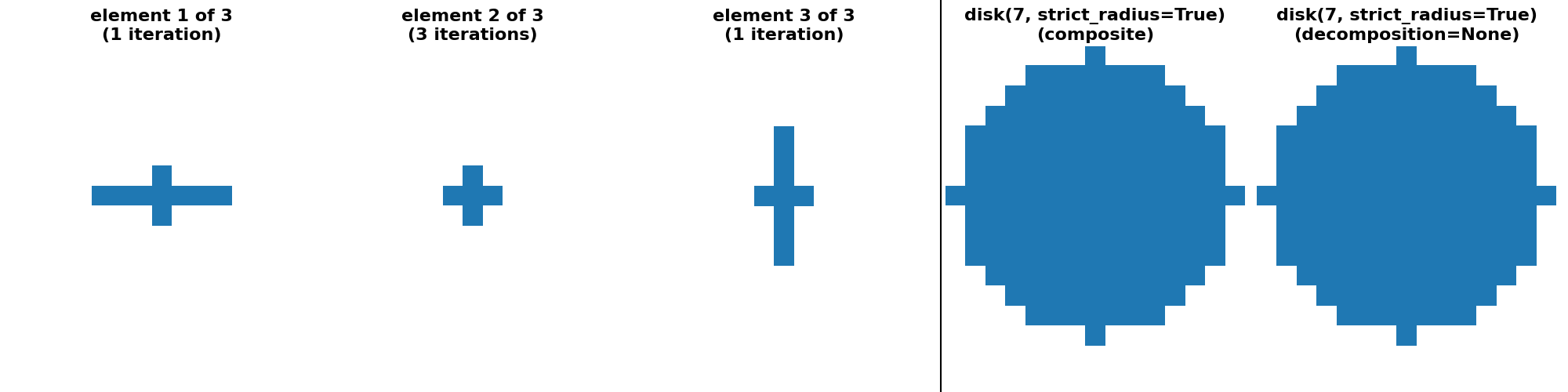•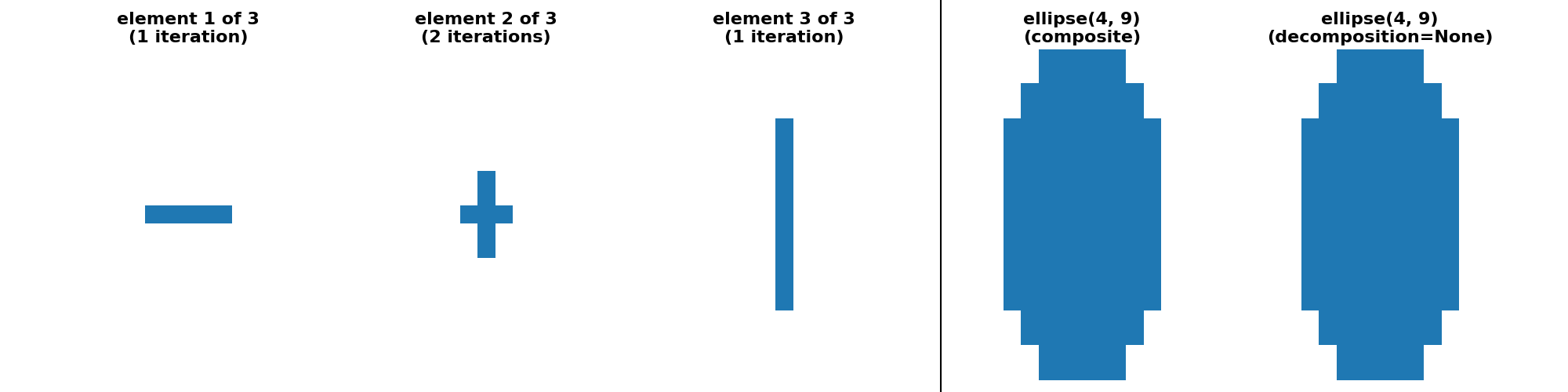•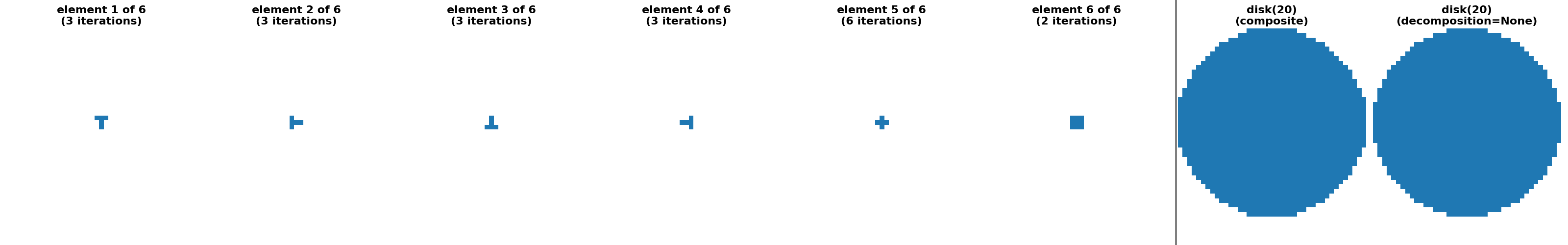•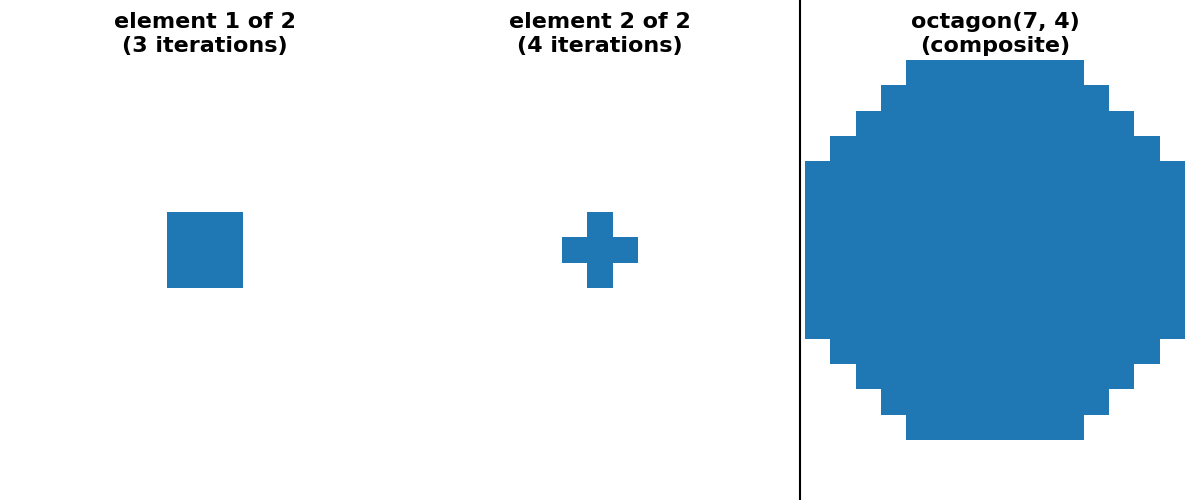•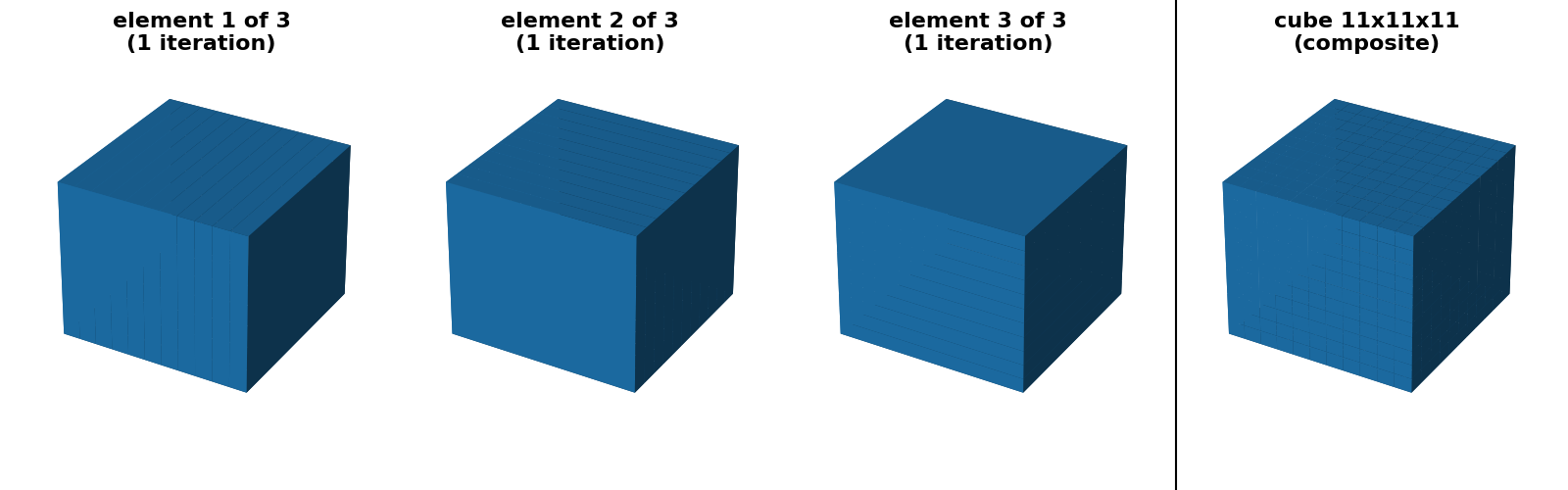•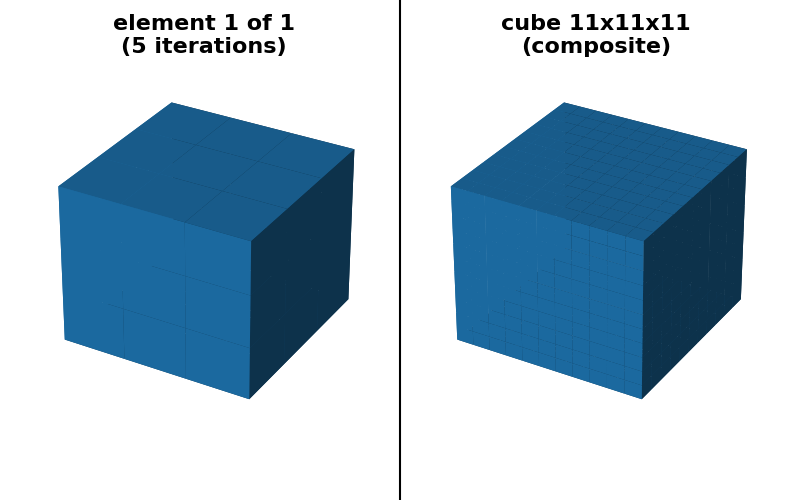•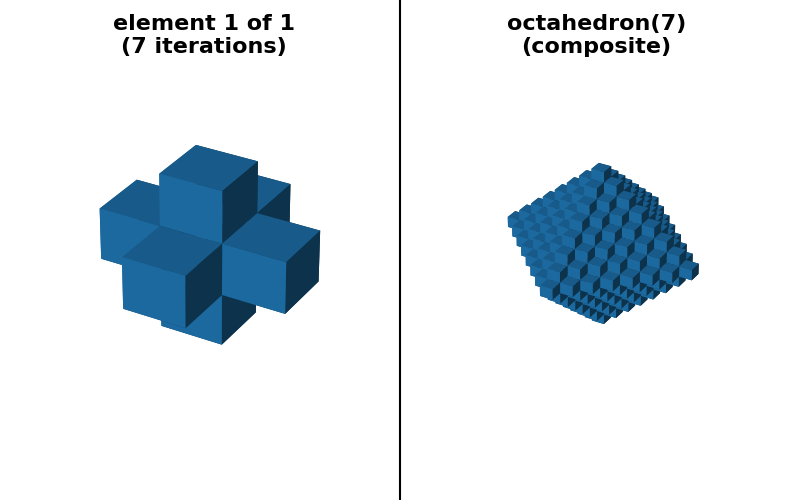•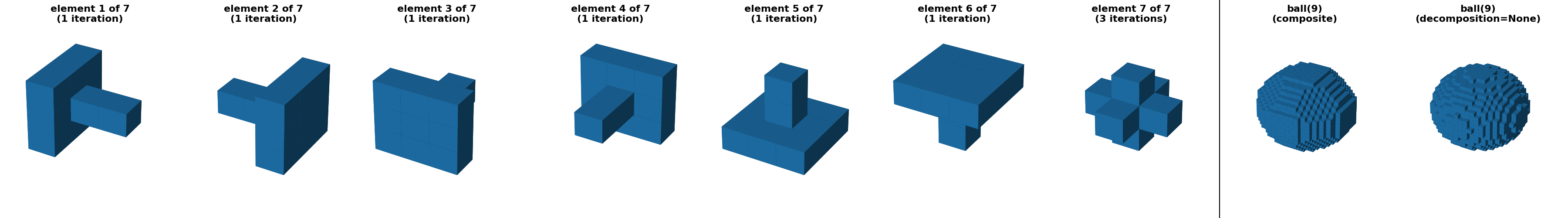```import numpy as np
import matplotlib.pyplot as plt
from matplotlib import colors
from mpl_toolkits.mplot3d import Axes3D

from skimage.morphology import (ball, cube, diamond, disk, ellipse, octagon,
octahedron, rectangle, square)
from skimage.morphology.footprints import footprint_from_sequence

# Generate 2D and 3D structuring elements.
footprint_dict = {
"square(11) (separable)": (square(11, decomposition=None),
square(11, decomposition="separable")),
"square(11) (sequence)": (square(11, decomposition=None),
square(11, decomposition="sequence")),
"rectangle(7, 11) (separable)": (rectangle(7, 11, decomposition=None),
rectangle(7, 11,
decomposition="separable")),
"rectangle(7, 11) (sequence)": (rectangle(7, 11, decomposition=None),
rectangle(7, 11,
decomposition="sequence")),
"diamond(5) (sequence)": (diamond(5, decomposition=None),
diamond(5, decomposition="sequence")),
),
),
"ellipse(4, 9) (crosses)": (
ellipse(4, 9, decomposition=None),
ellipse(4, 9, decomposition="crosses")
),
decomposition="sequence")),
"octagon(7, 4) (sequence)": (octagon(7, 4, decomposition=None),
octagon(7, 4, decomposition="sequence")),
"cube(11) (separable)": (cube(11, decomposition=None),
cube(11, decomposition="separable")),
"cube(11) (sequence)": (cube(11, decomposition=None),
cube(11, decomposition="sequence")),
"octahedron(7) (sequence)": (octahedron(7, decomposition=None),
octahedron(7, decomposition="sequence")),
decomposition="sequence")),
}

# Visualize the elements

# binary white / blue colormap
cmap = colors.ListedColormap(['white', (0.1216, 0.4706, 0.70588)])

fontdict = dict(fontsize=16, fontweight='bold')
for title, (footprint, footprint_sequence) in footprint_dict.items():
ndim = footprint.ndim
num_seq = len(footprint_sequence)
approximate_decomposition = (
'ball' in title or 'disk' in title or 'ellipse' in title
)
if approximate_decomposition:
# Two extra plot in approximate cases to show both:
# 1.) decomposition=None idea footprint
# 2.) actual composite footprint corresponding to the sequence
num_subplots = num_seq + 2
else:
# composite and decomposition=None are identical so only 1 extra plot
num_subplots = num_seq + 1
fig = plt.figure(figsize=(4 * num_subplots, 5))
if ndim == 2:
ax.imshow(footprint, cmap=cmap, vmin=0, vmax=1)
if approximate_decomposition:
ax2 = fig.add_subplot(1, num_subplots, num_subplots - 1)
footprint_composite = footprint_from_sequence(footprint_sequence)
ax2.imshow(footprint_composite, cmap=cmap, vmin=0, vmax=1)

else:
projection=Axes3D.name)
ax.voxels(footprint, cmap=cmap)
if approximate_decomposition:
ax2 = fig.add_subplot(1, num_subplots, num_subplots - 1,
projection=Axes3D.name)
footprint_composite = footprint_from_sequence(footprint_sequence)
ax2.voxels(footprint_composite, cmap=cmap)

title1 = title.split(' (')
if approximate_decomposition:
# plot decomposition=None on a separate axis from the composite
title = title1 + '\n(decomposition=None)'
else:
# for exact cases composite and decomposition=None are identical
title = title1 + '\n(composite)'
ax.set_title(title, fontdict=fontdict)
ax.set_axis_off()
if approximate_decomposition:
ax2.set_title(title1 + '\n(composite)', fontdict=fontdict)
ax2.set_axis_off()

for n, (fp, num_reps) in enumerate(footprint_sequence):
npad = [((footprint.shape[d] - fp.shape[d]) // 2, ) * 2
for d in range(ndim)]
if ndim == 2:
ax = fig.add_subplot(1, num_subplots, n + 1)
ax.imshow(fp, cmap=cmap, vmin=0, vmax=1)
else:
ax = fig.add_subplot(1, num_subplots, n + 1,
projection=Axes3D.name)
ax.voxels(fp, cmap=cmap)
title = f"element {n + 1} of {num_seq}\n({num_reps} iteration"
title += "s)" if num_reps > 1 else ")"
ax.set_title(title, fontdict=fontdict)
ax.set_axis_off()
ax.set_xlabel(f'num_reps = {num_reps}')
fig.tight_layout()

# draw a line separating the sequence elements from the composite
line_pos = num_seq / num_subplots
line = plt.Line2D([line_pos, line_pos], [0, 1], color="black")